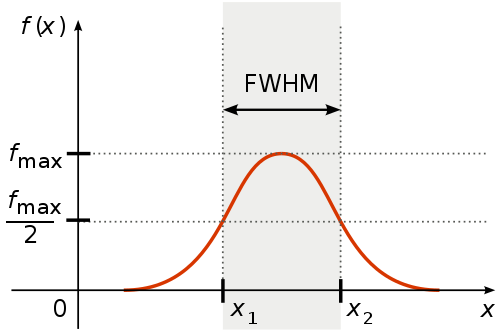# FWHM

Full width at half maximum##### Full width at half maximum

Concept in statistics and wave theory

In a distribution, full width at half maximum (FWHM) is the difference between the two values of the independent variable at which the dependent variable is equal to half of its maximum value. In other words, it is the width of a spectrum curve measured between those points on the y-axis which are half the maximum amplitude. Half width at half maximum (HWHM) is half of the FWHM if the function is symmetric. The term full duration at half maximum (FDHM) is preferred when the independent variable is time.